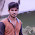## Pages

### Program for printing the Upper and Lower triangular matrices of a square matrix (for Plus2 Students)

```#include<iostream.h>
#include<conio.h>
#include<process.h>
void l_triangle(int a, int n)
{
int i=0, j=0;
for(i=0;i<n;i++)
{
for(j=0;j<n;j++)
{
if(i>=j)
cout<<a[i][j]<<" ";
else
cout<<" ";
}
cout<<"\n";
}
}
void u_triangle(int a, int n)
{
int i=0, j=0;
for(i=0;i<n;i++)
{
for(j=0;j<n;j++)
{
if(i<=j)
cout<<a[i][j]<<" ";
else
cout<<" ";
}
cout<<"\n";
}
}
void main()
{
clrscr();
int i, j, a, r, c; char ch;
do
{
cout<<"\n\nMENU\n\n";
cout<<"1. Enter elements\n2. Display matrix"
<<"\n3. Display upper triangle\n4. Display lower triangle"
<<"\n5. Exit";
cout<<"\nEnter your choice ";
cin>>c;
switch(c)
{
case 1: cout<<"\nEnter row and column(square matrix only)";
cin>>r>>c;
cout<<"\nEnter the elements";
for(i=0;i<r;i++)
{for(j=0;j<c;j++)
cin>>a[i][j];
}
break;
case 2: cout<<"\nMatrix is";
for(i=0;i<r;i++)
{
cout<<"\n";
for(j=0;j<r;j++)
cout<<a[i][j]<<" ";
}
break;
case 3: cout<<"\nUpper triangle matrix\n";
u_triangle(a,r);
break;
case 4: cout<<"\nLower triangle matrix\n";
l_triangle(a,r);
break;
case 5: cout<<"\nExit";
exit(0);
default: cout<<"\nWrong choice";
}
cout<<"\nDo you want to continue";
cin>>ch;
}while( ch=='Y'|| ch=='y');
getch();
}
```

#### 2 comments:

1.Nice and very helpful information i have got from your post : click here

2.In what language is it written? For upper triangular matrix, if column position is smaller than row position we simply make that position 0? Please help, don't let me postpone it any later so it gets to writersperhour.com, and their review is so not favorable.